Construction of Angles

Constructing 30, 60, 90, 120 Degree Angles

One of the most important aspects of geometry is constructing angles. Understanding the basics of this is very important and thus one must try and learn this properly.

• 1. Constructing a 60-degree angle

• In an equilateral triangle, all the angles are equal and they are equal to 60º in size. Thus, in order to draw 60º, we need to construct an equilateral triangle.

Step 1: Draw the arm PQ
Step 2: Then place the point of the compass at P and draw an arc such that it passes through Q
Step 3: Now place the point of the compass at Q and draw an arc such that it passes through P. The arc drawn at this step should cut the arc drawn in the above step at R.
Step 4: Now joining P to R. This angle measures 60º as the triangle PQR formed is an equilateral triangle.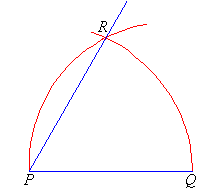• 2. Constructing a 30º angle

• We know that 30º is half of 60º. So we can apply this knowledge to construct a 30º angle.

Step 1: In order to construct an angle of 30º, we first need to construct an angle of 60º and then further bisect it.
Step 2: Draw the arm PQ
Step 3: Then place the point of the compass and then draw an arc which passes through Q.
Step 4: Place the point of the compass at Q and then draw the arc which cuts the arc drawn at the above step at another point R.
Step 5: WIth the compass at Q, draw another arc near the point T as shown in the figure.
Step 6: Now with the point of the compass at R, draw another arc in order to cut the arc drawn at step 5 at T.
Step 6: Now join the point T to the point P. Now the angle QPT is 30º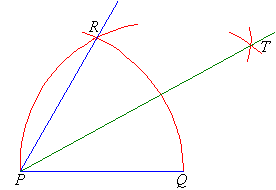• 3. Constructing a 120º angle

• 120º angle can be constructed using the logic that 60º + 120º = 180º. Thus, we can understand that in order to construct 120º we can construct 60º angle and then further extend one of its arms as shown below in the figure.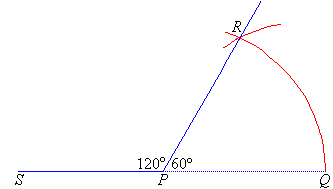• 4. Constructing a 90º angle

• In order to construct a 90º angle, there are two ways. The first one is by bisecting a straight angle and the other step is given below.

Step 1: First draw the arm PA.
Step 2: Now place the point of the compass at P and then draw an arc which cuts the arm at Q.
Step 3: Now place the point of the compass at Q and draw an arc which has the radius similar to the radius of PQ. This arc must cut the arc which is drawn in the above step at a point R.
Step 4: Now place the point of the compass at R and draw an arc of the radius which is similar to the radius of PQ in order to cut the arc which is drawn at step 2 at another point S.
Step 5: With the point of the compass at R, draw another arc of radius the same as the radius of PQ near the point T as shown in the figure below.
Step 6: With the point of the compass at S, draw an arc of radius similar to the radius of arc PQ and cut the arc which was drawn at step 5 at the point T.
Step 6: Join T to P using a scale. The angle APT formed measures 90º.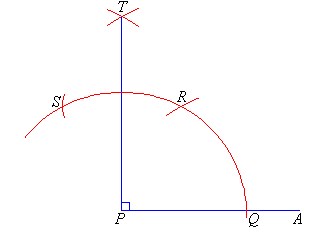Example problem

Use a ruler and compass and construct a triangle ABC. in the triangle, AB = 5 cm, angle BAC = 60º and AC = 4.5 cm.
Also, measure the angle of angle ABC and angle ACB and calculate the angle sum of ABC

Solution
Step 1: First draw the line AB which is 6m long.
Step 2: Now use a compass to construct a 60º angle at point A.
Step 3: Use the ruler to find C such that AC is 4.5 cm long.
Step 4: Now, join B to C.

The triangle ABC formed is the required triangle.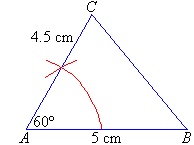Now, for the next part, we can use a protractor to find the rest of the angles.

Thus we can conclude that,
Angle ABC = 55º
Angle ACB = 65º
Thus, the angle sum of the triangle ABC = 60º + 55º + 65º = 180º